Share

Selina solutions for Class 10 Mathematics chapter 16 - Loci (Locus and Its Constructions)

Selina ICSE Concise Mathematics for Class 10 (2018-2019)

Selina Selina ICSE Concise Mathematics Class 10 (2018-2019)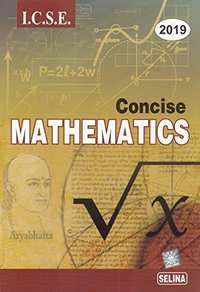Chapter 16: Loci (Locus and Its Constructions)

16A16B

Chapter 16: Loci (Locus and Its Constructions) Exercise 16A solutions [Page 0]

Given: PQ is perpendicular bisector of side AB of the triangle ABC.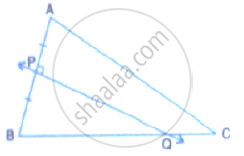Prove: Q is equidistant from A and B.

Given: CP is bisector of angle C of ΔABC.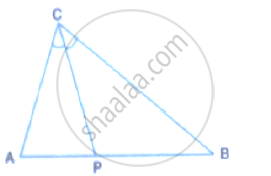Prove: P is equidistant from AC and BC.

Given: AX bisects angle BAC and PQ is perpendicular bisector of AC which meets AX at point Y.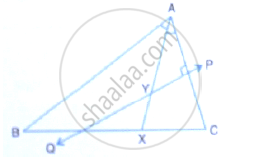Prove: (i) X is equidistant from AB and AC.
(ii) Y is equidistant from A and C.

Construct a triangle ABC, in which AB = 4.2 cm, BC = 6.3 cm and AC = 5cm. Draw perpendicular bisector of BC which meets AC at point D. Prove that D is equidistant from B and C.

In each of the given figures; PA = PB and QA = QB.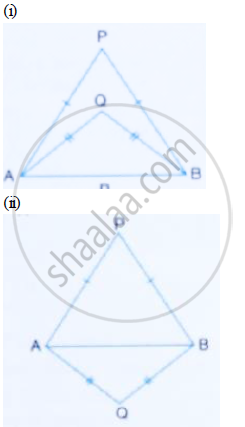Prove, in each case, that PQ (produce, if required) is perpendicular bisector of AB. Hence, state the locus of the points equidistant from two given fixed points.

Construct a right angled triangle PQR, in which ∠Q = 90°, hypotenuse PR = 8 cm and QR = 4.5 cm. Draw bisector of angle PQR and let it meets PR at point t. Prove that T is equidistant from PQ and QR.

Construct a triangle ABC in which angle ABC = 75°, AB= 5cm and BC =6.4cm. Draw perpendicular bisector of side BC and also the bisector of angle ACB. If these bisectors intersect each other at point P; prove that P is equidistant from B and C; and also from AC and BC.

In parallelogram ABCD, side AB is greater than side BC and P is a point in AC such that PB bisects angle B.
Prove that P is equidistant from AB and BC.

In triangle LMN, bisectors of interior angles at L and N intersect each other at point A. prove that:
(i) Point A is equidistant from all the three sides of the triangle.
(ii) AM bisects angle LMN.

Use ruler and compasses only for this question.
(i) Construct ΔABC, where AB = 3.5 cm, BC = 6cm and ∠ABC = 60°.
(ii) Construct the locus of points inside the triangle which are equidistant from BA and BC.
(iii) Construct the locus of points inside the triangle which are equidistant from B and C.
(iv) Mark the point P which is equidistant from AB, BC and also equidistant from B and C. measure and record the length of PB.

The given figure shows a triangle ABC in which AD bisects angle BAC. EG is perpendicular bisector of side AB which intersects AD at point F.
Prove that: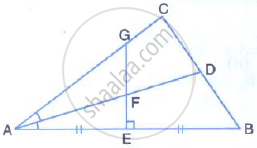F is equidistant from A and B.

The given figure shows a triangle ABC in which AD bisects angle BAC. EG is perpendicular bisector of side AB which intersects AD at point F.
Prove that: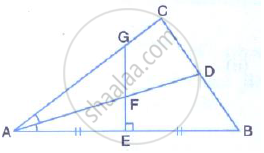(ii) F is equidistant from AB and AC.

The bisectors of ∠B and ∠C of a quadrilateral ABCD intersect each other at point P. Show that P is equidistant from the opposite sides AB and CD.

Draw a line AB = 6 cm. Draw the locus of all the points which are equidistant from A and B.

Draw an angle ABC = 75° Draw the locus of all the points equidistant from AB and BC.

Draw an ∠ABC = 60°, having AB = 4.6 cm and BC = 5cm. Find a point P equidistant from AB and BC; and also equidistant from A and B.

In the figure given below, find a point P on CD equidistant from points A and B.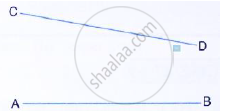In the given triangle ABC, find a point P equidistant from AB and AC; and also equidistant from B and C.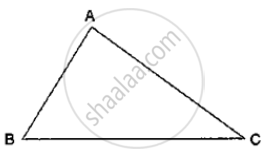Construct a triangle ABC, with AB = 7cm, BC = 8cm and ∠ABC = 60°. Locate by construction the point P such that:
(i) P is equidistant from B and C.
(ii) P is equidistant from AB and BC.
Measure and record the length of PB.

On a graph paper, draw the lines x = 3 and y = - 5. Now, on the same graph paper, draw the locus of the point which is equidistant from the given lines.

On a graph paper, draw the line x = 6. Now, on the same graph paper, draw the locus of the point which moves in such a way that its distantce from the given line is always equal to 3 units

Chapter 16: Loci (Locus and Its Constructions) Exercise 16B solutions [Page 0]

Describe the locus for questions 1 to 13 given below:
1. The locus of a point at a distant 3 cm from a fixed point.

The locus of points at a distance 2cm from a fixed line.

The locus of the centre of a wheel of a bicycle going straight along a level road.

The locus of the moving end of the minute hand of a clock.

The locus of a stone dropped from the top of a tower.

The locus of a runner, running round a circular track and always keeping a distance of 1.5 m from the inner edge.

The locus of the door handle, as the door opens .

The locus of points inside a circle and equidistant from two fixed points on the circumference of the circle.

The locus of the centres of all circles passing through two fixed points.

The locus of vertices of all isosceles triangles having a common base.

The locus of a point in space, which is always at a distance of 4cm from a fixed point.

The locus of a point P, so that:
AB^2 = AP^2 + BP^2,
Where A and B are two fixed points.

The locus of a point in rhombus ABCD, so that it is equidistant from
(i) AB and BC; (ii) B  and D .

The speed of sound is 332 metres per second. A gun is fired. Describe the locus of all the people on the earth’s surface, who hear the sound exactly one second later.

Describe:

The locus of points at distances less than 3cm from a given point.

Describe:

The locus of points at distances greater than 4 cm from a given point.

Describe:

The locus of points at distances less than or equal to 2.5 cm from a given point.

Describe:

The locus of points at distances greater than or equal to 35 mm from a given point.

Describe:

The locus of the centres of a given circle which rolls around the outside of a second circle and is always touching it.

Describe:

The locus of the centres of all circles that are tangent to both the arms of a given angle.

Describe:

The locus of the mod-points of all chords parallel to a given chord of a circle.

Describe:

The locus of points within a circle that are equidistant from the end points of a given chord.

Sketch and describe the locus of the vertices of all triangles with a given base and a given altitude.

In the given figure, obtain all the points equidistant from lines m and n; and 2.5 cm from O.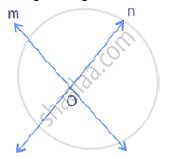By actual drawing obtain the points equidistant from lines m and n; and 6 cm from a point P, where P is 2 cm above m, m is parallel to n and m is 6 cm above n.

A straight line AB is 8cm long. Draw and describe the locus of a point which is:
(i) always 4 cm from the line AB.
(ii) equidistant from A and B.
Mark the two points X and Y, which are 4cm from AB and equidistant from A and B. describe the figure AXBY.

Angle ABC = 60° and BA = BC = 8 cm. The mid points of BA and BC are M and N respectively. Draw and describe the locus of a point which is:
(i) Equidistant from BA and BC.
(ii) 4 cm from M
(iii) 4 cm from N
Mark the point P, which is 4 cm from both M and N, and equidistant from BA and BC. Join MP and NP, and describe the figure BMPN.

Draw a triangle ABC in which AB = 6cm, BC = 4.5 cm and AC = 5cm. Draw and label:
(i) the locus of the centres of all circles which touch AB and AC,
(ii) the locus of the centres of all the circles of radius 2 cm which touch AB.
Hence, construct the circle of radius 2cm which touches AB and AC .

Construct a triangle ABC, having given AB = 4.8 cm, AC = 4cm, and ∠A = 75°.
Find a point P.
(i) Inside the triangle ABC.
(ii) outside the triangle ABC
Equidistant from B and C; and at a distance of 1.2 cm from BC.

O is a fixed point. Point P moves along a fixed line AB. Q is a point on OP produced such that OP = PQ. Prove that the locus of point Q is a line parallel to AB.

Draw an angle ABC = 75°. Find a point P such that P is at a distance of 2 cm from AB and 1.5 cm from BC.

Construct a triangle ABC, with AB = 5.6 cm, AC = BC = 9.2 cm. Find the points equidistant from AB and AC; and also 2 cm from BC. Measure the distance between the two points obtained.

Construct a triangle ABC, with AB = 6 cm, AC = BC = 9cm. Find a point 4 cm from A and equidistant from B and C.

Ruler and compasses may be used in this question. All construction lines and arcs must be clearly shown and be of sufficient length and clarity to permit assessment.
(i) Construct a ΔABC, in which BC = 6cm, AB = 9 cm and angle ABC = 60°.
(ii) Construct the locus of all points inside triangle ABC, which are equidistant from B and C.
(iii) Construct the locus of the vertices of the triangles with BC as base and which are equal in area to triangle ABC.
(iv) Mark the point Q, in your construction, which would make ΔQBC equal in area to ΔABC, and isosceles.
(v) Measure and record the length of CQ.

State the locus of a point in a rhombus ABCD, which is equidistant
(i) from AB and AD;
(ii) from the vertices A and C.

Use graph paper for this question. Take 2 cm = 1 unit on both the axes.
(i) Plot the points A(1,1), B(5,3) and C(2,7).
(ii) Construct the locus of points equidistant from A and B.
(iii) Construct the locus of points equidistant from AB and AC.
(iv) locate the point P such that PA = PB and P is equidistant from AB and AC.
(v) Measure and record the length PA in cm.

Construct an isosceles triangle ABC such that AB = 6cm, BC = AC = 4cm. Bisect ∠C internally and mark a point P on this bisector such that CP = 5 cm. Find the points Q and R which are 5 cm from P and also 5 cm from the line AB.

Use ruler and compasses only for this question. Draw a circle of radius 4 cm and mark two chords AB and AC of the circle of lengths 6 cm and 5 cm respectively.
(i) Construct the locus of points, inside the circle, that are equidistant from A and C. prove your construction.
(ii) Construct the locus of points, inside the circle that are equidistant from AB and AC.

Plot the points A(2, 9), B(-1, 3) and C (6, 3) on graph paper. On the same graph paper draw the locus of point A so that the area of ΔABC remains the same as A moves.

Construct a triangle BCP given BC = 5cm, BP = 4cm and ∠PBC = 45°.
(i) Complete the rectangle ABCD such that:
(a) P is equidistant from AB and BC.
(b) P is equidistant from C and D.
(ii) Measure and record the length of AB.

Chapter 16: Loci (Locus and Its Constructions)

16A16B

Selina Selina ICSE Concise Mathematics Class 10 (2018-2019)Selina solutions for Class 10 Mathematics chapter 16 - Loci (Locus and Its Constructions)

Selina solutions for Class 10 Maths chapter 16 (Loci (Locus and Its Constructions)) include all questions with solution and detail explanation. This will clear students doubts about any question and improve application skills while preparing for board exams. The detailed, step-by-step solutions will help you understand the concepts better and clear your confusions, if any. Shaalaa.com has the CISCE Selina ICSE Concise Mathematics for Class 10 (2018-2019) solutions in a manner that help students grasp basic concepts better and faster.

Further, we at Shaalaa.com are providing such solutions so that students can prepare for written exams. Selina textbook solutions can be a core help for self-study and acts as a perfect self-help guidance for students.

Concepts covered in Class 10 Mathematics chapter 16 Loci (Locus and Its Constructions) are Introduction of Loci, Loci Examples, Constructions Under Loci, Theorems Based on Loci.

Using Selina Class 10 solutions Loci (Locus and Its Constructions) exercise by students are an easy way to prepare for the exams, as they involve solutions arranged chapter-wise also page wise. The questions involved in Selina Solutions are important questions that can be asked in the final exam. Maximum students of CISCE Class 10 prefer Selina Textbook Solutions to score more in exam.

Get the free view of chapter 16 Loci (Locus and Its Constructions) Class 10 extra questions for Maths and can use Shaalaa.com to keep it handy for your exam preparation

S